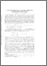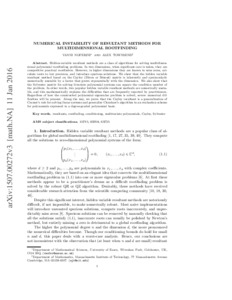# Numerical instability of resultant methods for multidimensional rootfinding

Noferini, V and Townsend, A (2016) 'Numerical instability of resultant methods for multidimensional rootfinding.' SIAM Journal on Numerical Analysis, 54 (2). 719 - 743. ISSN 0036-1429Preview
Text
1507.00272v3.pdf

Download (380kB) | Preview

## Abstract

Hidden-variable resultant methods are a class of algorithms for solving multidimensional polynomial rootfinding problems. In two dimensions, when significant care is taken, they are competitive practical rootfinders. However, in higher dimensions they are known to miss zeros, calculate roots to low precision, and introduce spurious solutions. We show that the hidden variable resultant method based on the Cayley (Dixon or Bézout) matrix is inherently and spectacularly numerically unstable by a factor that grows exponentially with the dimension. We also show that the Sylvester matrix for solving bivariate polynomial systems can square the condition number of the problem. In other words, two popular hidden variable resultant methods are numerically unstable, and this mathematically explains the difficulties that are frequently reported by practitioners. Regardless of how the constructed polynomial eigenvalue problem is solved, severe numerical difficulties will be present. Along the way, we prove that the Cayley resultant is a generalization of Cramer's rule for solving linear systems and generalize Clenshaw's algorithm to an evaluation scheme for polynomials expressed in a degree-graded polynomial basis.

Item Type: Article Q Science > QA Mathematics Faculty of Science and Health > Mathematical Sciences, Department of Jim Jamieson 27 May 2016 13:35 31 Mar 2021 07:15 http://repository.essex.ac.uk/id/eprint/16822

### Actions (login required)View Item